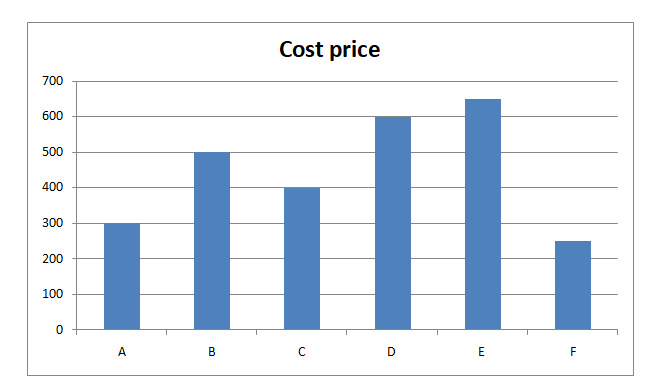# LIC AAO/SBI PO Prelims Quantitative Aptitude Questions 2019 (Day-24)

Dear Aspirants, Our IBPS Guide team is providing new series of Quantitative Aptitude Questions for LIC AAO/SBI PO 2019 so the aspirants can practice it on a daily basis. These questions are framed by our skilled experts after understanding your needs thoroughly. Aspirants can practice these new series questions daily to familiarize with the exact exam pattern and make your preparation effective.

[WpProQuiz 5936]

### Click Here for SBI PO Pre 2019 High-Quality Mocks Exactly on SBI Standard

Directions (Q. 1 – 5): In the following questions, two equations I and II are given. You have to solve both the equations and give answer as,

a) If x > y

b) If x ≥ y

c) If x < y

d) If x ≤ y

e) If x = y or the relation cannot be established

1)

I) 8x – 3y = -4

II) 9x – 4y = -2

2)

I) x2 + 5x – 126 = 0

II) y2 – 4y – 77 = 0

3)

I) x3 + (156 ÷ 4) – 12 % of 50 = 60

II) y2 – 12y + 27 = 0

4)

I) 2x2 – 5x – 33 = 0

II) y2 – 17y + 66 = 0

5)

I) x2 = 140608 + (3/35) of 140

II) y3 = 729

Directions (Q. 6 – 10) Study the following information carefully and answer the given questions:

The following bar graph shows the cost price of 6 different items.6) Find the ratio between the marked price of item A to the cost price of item C, if the mark-up % of item A is 25 %?

a) 23 : 27

b) 19 : 22

c) 37 : 45

d) 15 : 16

e) None of these

7) Find the average cost price of item B, D and F together?

a) Rs. 450

b) Rs. 420

c) Rs. 380

d) Rs. 540

e) None of these

8) Find the difference between the selling price of item C to that of item E, if the profit % of item C is 20 % and loss % of item E is 10 %?

a) Rs. 70

b) Rs. 145

c) Rs. 105

d) Rs. 180

e) None of these

9) Find the approximate profit % of item P, if the cost price of item P is 75 % of cost price of item D and the selling price of item P is equal to the cost price of item B?

a) 7 % loss

b) 11 % profit

c) 18 % profit

d) 15 % loss

e) 23 % profit

10) The cost price of item A and E together is approximately what percentage of the cost price of item D and F together?

a) 95 %

b) 78 %

c) 143 %

d) 112 %

e) 130 %

8x – 3y = -4 –> (1)

9x – 4y = -2 –> (2)

By solving the equation (1) and (2), we get,

X = -2, y = -4

X > y

I) x2 + 5x – 126 = 0

(x – 9) (x + 14) = 0

X = 9, -14

II) y2 – 4y – 77 = 0

(y – 11) (y + 7) = 0

Y = 11, -7

Can’t be determined

I) x3 + (156 ÷ 4) – 12 % of 50 = 60

X3 + (156/4) – (12/100)*50 = 60

X3 = 60 – 39 + 6

X3 = 27

X = 3

II) y2 – 12y + 27 = 0

(y – 9) (y – 3) = 0

Y = 9, 3

X ≤ y

I) 2x2 – 5x – 33 = 0

2x2 + 6x – 11x – 33 = 0

2x (x + 3) – 11 (x + 3) = 0

(2x – 11) (x + 3) = 0

X = 11/2, -3 = 5.5, -3

II) y2 – 17y + 66 = 0

(y – 11) (y – 6) = 0

Y = 11, 6

X < y

I) x2 = ∛140608 + (3/35)*140

X2 = 52 + 12 = 64

X = 8, -8

II) y3 = 729

Y = 9

X < y

The marked price of item A

= > 300*(125/100) = Rs. 375

The cost price of item C = Rs. 400

Required ratio = 375 : 400 = 15 : 16

The average cost price of item B, D and F together

= > (500 + 600 + 250)/3

= > 1350/3 = Rs. 450

The selling price of item C = 400*(120/100) = Rs. 480

The selling price of item E = 650*(90/100) = Rs. 585

Required difference = 585 – 480 = Rs. 105

The cost price of item P

= > 75 % of cost price of item D

= > (75/100)*600 = Rs. 450

The selling price of item P = The cost price of item B = Rs. 500

Profit % = [(500 – 450)/450]*100 = 11.11 % = 11 % profit

The cost price of item A and E together

= > 300 + 650 = Rs. 950

The cost price of item D and F together

= > 600 + 250 = Rs. 850

Required % = (950/850)*100 = 111.76 % = 112 %